# What is general relativity?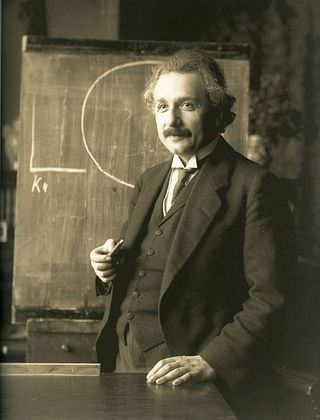David Tong (with the Plus team) in Plus Magazine:

The general theory of relativity describes the force of gravity. Einstein wasn't the first to come up with such a theory — back in 1686 Isaac Newton formulated his famous inverse square law of gravitation. Newton's law works perfectly well on small-ish scales: we can use it to calculate how fast an object dropped off a tall building will hurtle to the ground and even to send people to the Moon. But when distances and speeds are very large, or very massive objects are involved, Newton's law becomes inaccurate. It's a good place to start though, as it's easier to describe than Einstein's theory.

Suppose you have two objects, say the Sun and the Earth, with masses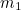and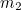respectively. Write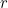for the distance between the two objects. Then Newton’s law says that the gravitational force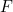between them is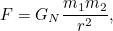where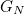is a fixed number, known as Newton's constant.

The formula makes intuitive sense: it tells us that gravity gets weaker over long distances (the largerthe smaller) and that the gravitational force is stronger between more massive objects (the larger either ofandthe larger).

Different force, same formula

There is another formula which looks very similar, but describes a different force. In 1785 the French physicist Charles-Augustin de Coulomb came up with an equation to capture the electrostatic forcethat acts between two charged particles with chargesand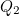: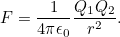Herestands for the distance between the two particles and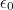is a constant which determines the strength of electromagnetism. (It has the fancy name permittivity of free space.)

More here.